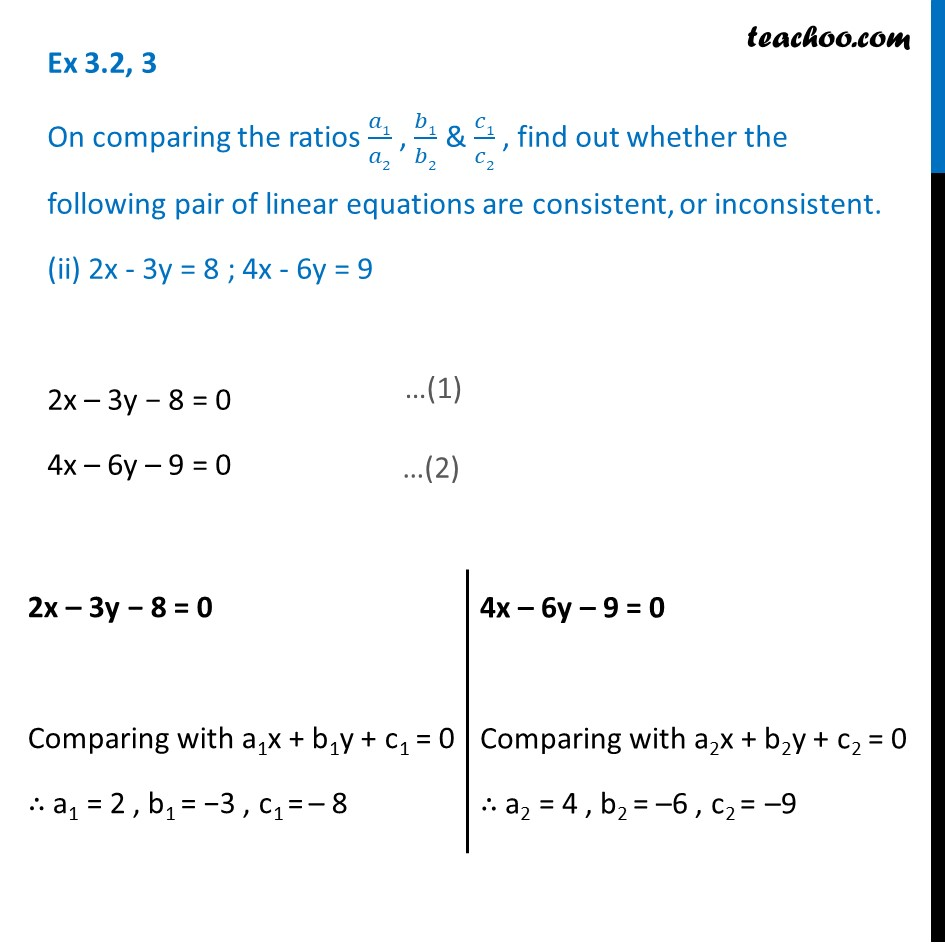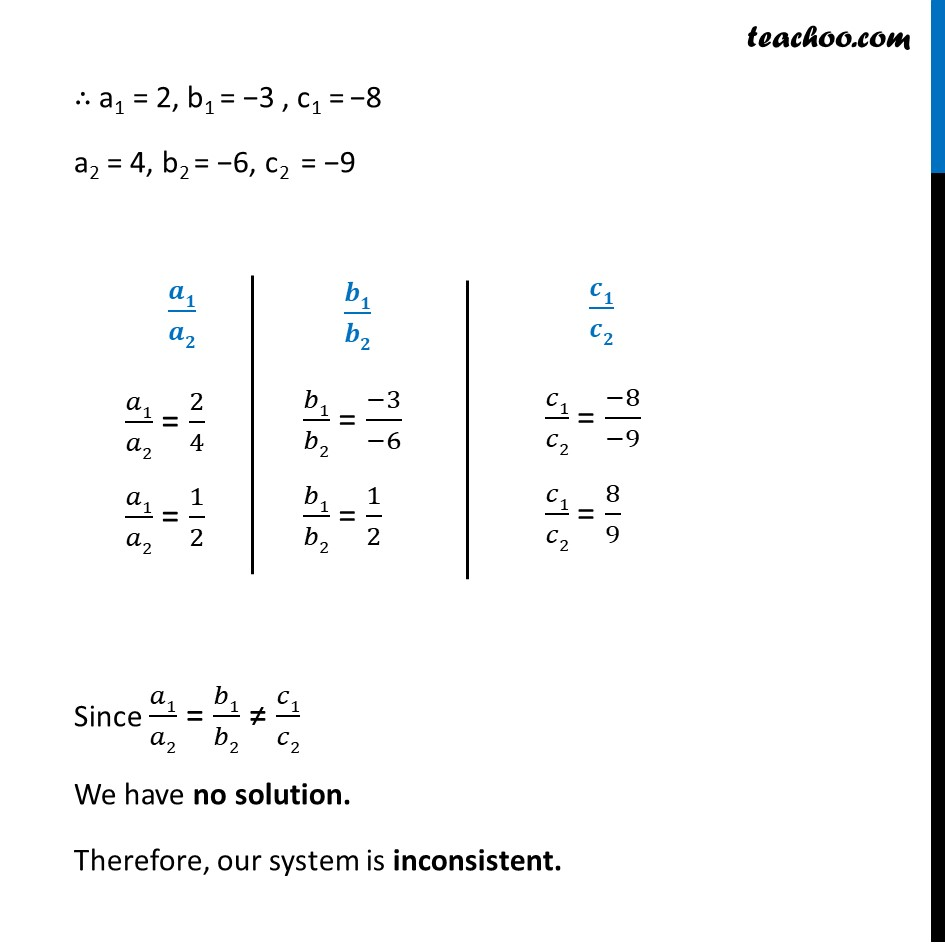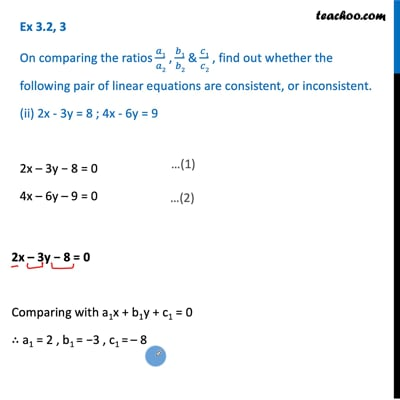Ex 3.2

Chapter 3 Class 10 Pair of Linear Equations in Two Variables
Serial order wiseThis video is only available for Teachoo black users

Maths Crash Course - Live lectures + all videos + Real time Doubt solving!

### Transcript

Ex 3.2, 3 On comparing the ratios 𝑎1/𝑎2 , 𝑏1/𝑏2 & 𝑐1/𝑐2 , find out whether the following pair of linear equations are consistent, or inconsistent. (ii) 2x - 3y = 8 ; 4x - 6y = 9 2x – 3y − 8 = 0 4x – 6y – 9 = 0 2x – 3y − 8 = 0 Comparing with a1x + b1y + c1 = 0 ∴ a1 = 2 , b1 = −3 , c1 = – 8 4x – 6y – 9 = 0 Comparing with a2x + b2y + c2 = 0 ∴ a2 = 4 , b2 = –6 , c2 = –9 ∴ a1 = 2, b1 = −3 , c1 = −8 a2 = 4, b2 = −6, c2 = −9 𝒂𝟏/𝒂𝟐 𝑎1/𝑎2 = 2/4 𝑎1/𝑎2 = 1/2 𝒃𝟏/𝒃𝟐 𝑏1/𝑏2 = (−3)/(−6) 𝑏1/𝑏2 = 1/2 𝒄𝟏/𝒄𝟐 𝑐1/𝑐2 = (−8)/(−9) 𝑐1/𝑐2 = 8/9 Since 𝑎1/𝑎2 = 𝑏1/𝑏2 ≠ 𝑐1/𝑐2 We have no solution. Therefore, our system is inconsistent.